# Introduction into scalable graph analysis with Apache Giraph and Spark GraphX

Jul. 24, 2015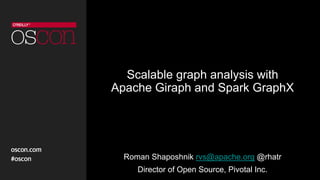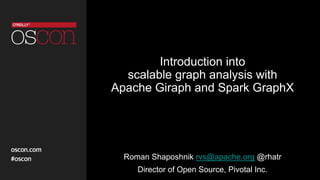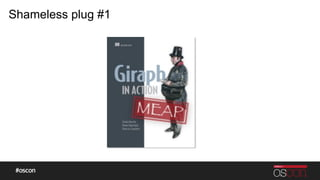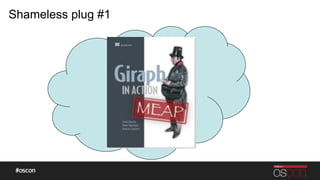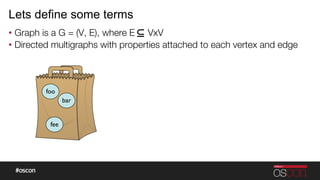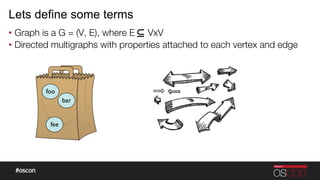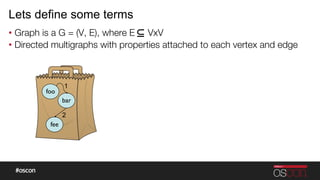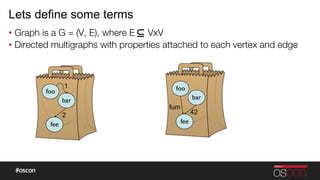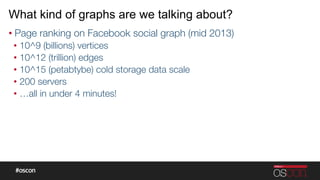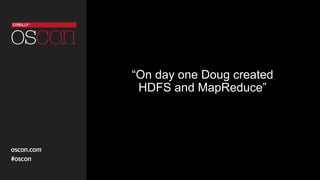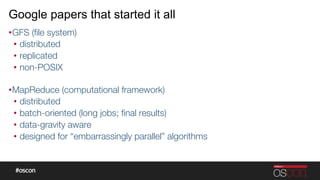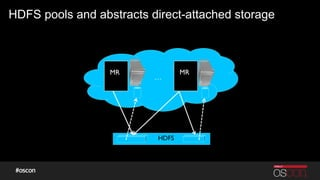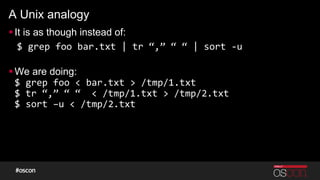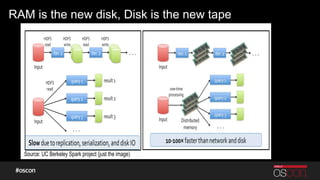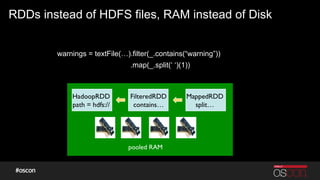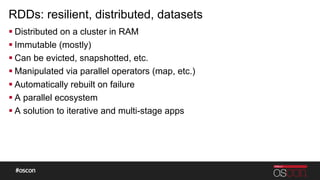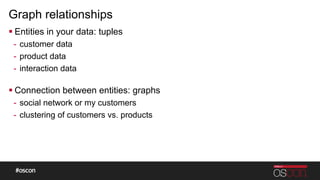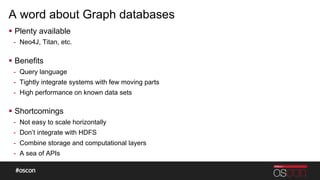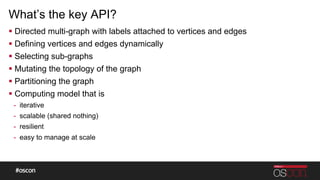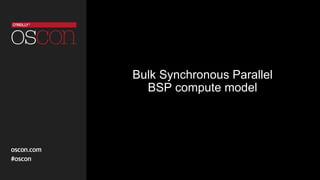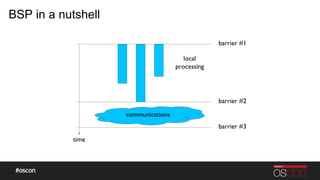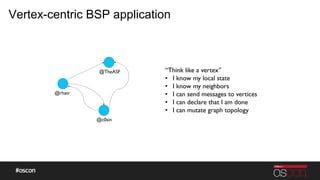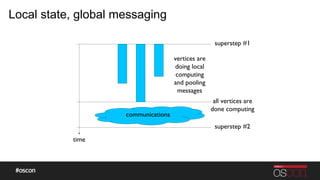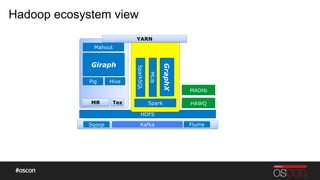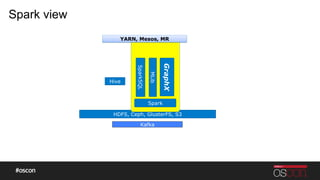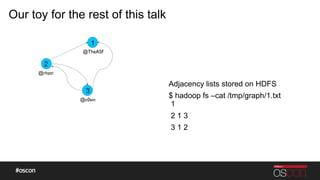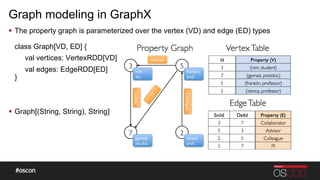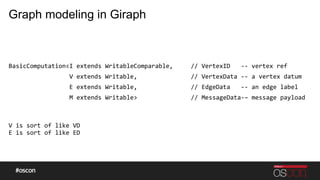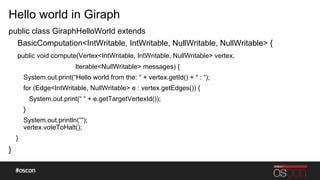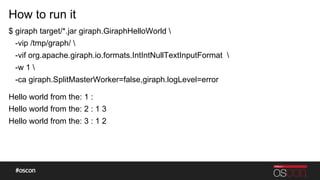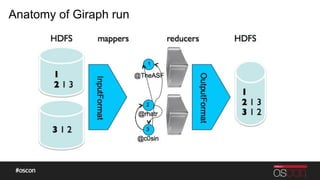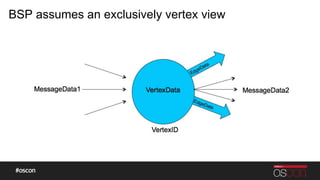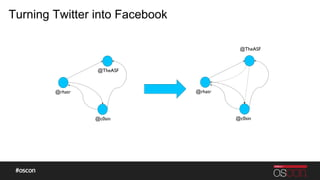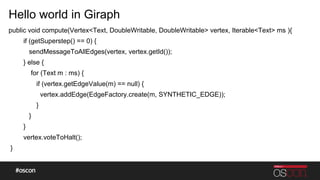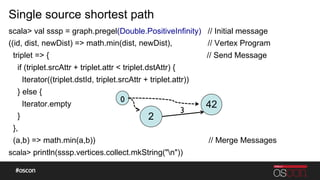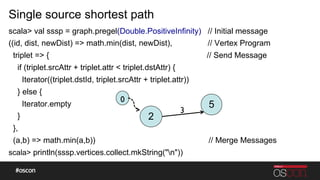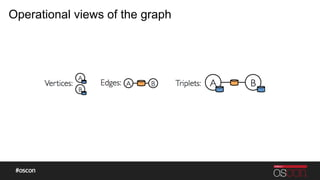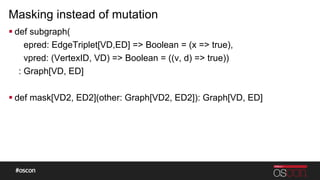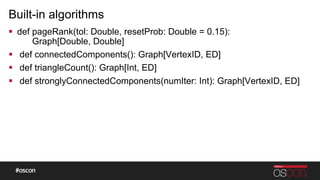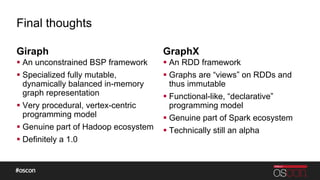1 of 48

### Introduction into scalable graph analysis with Apache Giraph and Spark GraphX

• 1. Scalable graph analysis with Apache Giraph and Spark GraphX Roman Shaposhnik rvs@apache.org @rhatr Director of Open Source, Pivotal Inc.
• 2. Introduction into scalable graph analysis with Apache Giraph and Spark GraphX Roman Shaposhnik rvs@apache.org @rhatr Director of Open Source, Pivotal Inc.
• 3. Shameless plug #1
• 4. Shameless plug #1
• 5. Agenda:
• 6. Lets define some terms •  Graph is a G = (V, E), where E VxV •  Directed multigraphs with properties attached to each vertex and edge foo bar fee
• 7. Lets define some terms •  Graph is a G = (V, E), where E VxV •  Directed multigraphs with properties attached to each vertex and edge foo bar fee
• 8. Lets define some terms •  Graph is a G = (V, E), where E VxV •  Directed multigraphs with properties attached to each vertex and edge foo bar fee 2 1
• 9. Lets define some terms •  Graph is a G = (V, E), where E VxV •  Directed multigraphs with properties attached to each vertex and edge foo bar fee 2 1 foo bar fee 42 fum
• 10. What kind of graphs are we talking about? • Page ranking on Facebook social graph (mid 2013) •  10^9 (billions) vertices •  10^12 (trillion) edges •  10^15 (petabtybe) cold storage data scale •  200 servers •  …all in under 4 minutes!
• 11. “On day one Doug created HDFS and MapReduce”
• 12. Google papers that started it all • GFS (ﬁle system) •  distributed •  replicated •  non-POSIX" • MapReduce (computational framework) •  distributed •  batch-oriented (long jobs; ﬁnal results) •  data-gravity aware •  designed for “embarrassingly parallel” algorithms
• 13. HDFS pools and abstracts direct-attached storage … HDFS MR MR
• 14. A Unix analogy § It is as though instead of: \$  grep  foo  bar.txt  |  tr  “,”  “  “  |  sort  -­‐u     § We are doing: \$  grep  foo  <  bar.txt  >  /tmp/1.txt   \$  tr  “,”  “  “    <  /tmp/1.txt  >  /tmp/2.txt   \$  sort  –u  <  /tmp/2.txt
• 15. Enter Apache Spark
• 16. RAM is the new disk, Disk is the new tape Source: UC Berkeley Spark project (just the image)
• 17. RDDs instead of HDFS files, RAM instead of Disk warnings = textFile(…).filter(_.contains(“warning”)) .map(_.split(‘ ‘)(1)) HadoopRDD path = hdfs:// FilteredRDD contains… MappedRDD split… pooled RAM
• 18. RDDs: resilient, distributed, datasets § Distributed on a cluster in RAM § Immutable (mostly) § Can be evicted, snapshotted, etc. § Manipulated via parallel operators (map, etc.) § Automatically rebuilt on failure § A parallel ecosystem § A solution to iterative and multi-stage apps
• 19. What’s so special about Graphs and big data?
• 20. Graph relationships § Entities in your data: tuples -  customer data -  product data -  interaction data § Connection between entities: graphs -  social network or my customers -  clustering of customers vs. products
• 21. A word about Graph databases §  Plenty available -  Neo4J, Titan, etc. §  Benefits -  Query language -  Tightly integrate systems with few moving parts -  High performance on known data sets §  Shortcomings -  Not easy to scale horizontally -  Don’t integrate with HDFS -  Combine storage and computational layers -  A sea of APIs
• 22. What’s the key API? § Directed multi-graph with labels attached to vertices and edges § Defining vertices and edges dynamically § Selecting sub-graphs § Mutating the topology of the graph § Partitioning the graph § Computing model that is -  iterative -  scalable (shared nothing) -  resilient -  easy to manage at scale
• 23. Bulk Synchronous Parallel BSP compute model
• 24. BSP in a nutshell time communications local processing barrier #1 barrier #2 barrier #3
• 25. Vertex-centric BSP application @rhatr @TheASF @c0sin “Think like a vertex” •  I know my local state •  I know my neighbors •  I can send messages to vertices •  I can declare that I am done •  I can mutate graph topology
• 26. Local state, global messaging time communications vertices are doing local computing and pooling messages superstep #1 all vertices are done computing superstep #2
• 27. Lets put it all together
• 28. Hadoop ecosystem view HDFS Pig Sqoop Flume MR Hive Tez Giraph Mahout Spark SparkSQL MLib GraphX HAWQ Kafka YARN MADlib
• 29. Spark view HDFS, Ceph, GlusterFS, S3 Hive Spark SparkSQL MLib GraphX Kafka YARN, Mesos, MR
• 30. Enough boxology! Lets look at some code
• 31. Our toy for the rest of this talk Adjacency lists stored on HDFS \$ hadoop fs –cat /tmp/graph/1.txt 1 2 1 3 3 1 2 @rhatr @TheASF @c0sin 3 1 2
• 32. Graph modeling in GraphX §  The property graph is parameterized over the vertex (VD) and edge (ED) types class Graph[VD, ED] { val vertices: VertexRDD[VD] val edges: EdgeRDD[ED] } §  Graph[(String, String), String]
• 33. Hello world in GraphX \$ spark*/bin/spark-shell scala val inputFile = sc.textFile(“hdfs:///tmp/graph/1.txt”) scala val edges = inputFile.flatMap(s = { // “2 1 3” val l = s.split(t); // [ “2”, “1”, “3” ] l.drop(1).map(x = (l.head.toLong, x.toLong)) // [ (2, 1), (2, 3) ] }) scala val graph = Graph.fromEdgeTuples(edges, ) // Graph[String, Int] scala val result = graph.collectNeighborIds(EdgeDirection.Out).map(x = println(Hello world from the: + x._1 + : + x._2.mkString( )) ) scala result.collect() // don’t try this @home Hello world from the: 1 : Hello world from the: 2 : 1 3 Hello world from the: 3 : 1 2
• 34. Graph modeling in Giraph BasicComputationI  extends  WritableComparable,          //  VertexID      -­‐-­‐  vertex  ref                                                                                                        V  extends  Writable,                              //  VertexData  -­‐-­‐  a  vertex  datum                                    E  extends  Writable,                              //  EdgeData      -­‐-­‐  an  edge  label                                    M  extends  Writable                              //  MessageData-­‐–  message  payload       V  is  sort  of  like  VD   E  is  sort  of  like  ED
• 35. Hello world in Giraph public class GiraphHelloWorld extends BasicComputationIntWritable, IntWritable, NullWritable, NullWritable { public void compute(VertexIntWritable, IntWritable, NullWritable vertex, IterableNullWritable messages) { System.out.print(“Hello world from the: “ + vertex.getId() + “ : “); for (EdgeIntWritable, NullWritable e : vertex.getEdges()) { System.out.print(“ “ + e.getTargetVertexId()); } System.out.println(“”); vertex.voteToHalt(); } }
• 36. How to run it \$ giraph target/*.jar giraph.GiraphHelloWorld -vip /tmp/graph/ -vif org.apache.giraph.io.formats.IntIntNullTextInputFormat -w 1 -ca giraph.SplitMasterWorker=false,giraph.logLevel=error Hello world from the: 1 : Hello world from the: 2 : 1 3 Hello world from the: 3 : 1 2
• 37. Anatomy of Giraph run
• 38. BSP assumes an exclusively vertex view
• 39. Turning Twitter into Facebook @rhatr @TheASF @c0sin @rhatr @TheASF @c0sin
• 40. Hello world in Giraph public void compute(VertexText, DoubleWritable, DoubleWritable vertex, IterableText ms ){ if (getSuperstep() == 0) { sendMessageToAllEdges(vertex, vertex.getId()); } else { for (Text m : ms) { if (vertex.getEdgeValue(m) == null) { vertex.addEdge(EdgeFactory.create(m, SYNTHETIC_EDGE)); } } } vertex.voteToHalt(); }
• 41. BSP in GraphX
• 42. Single source shortest path scala val sssp = graph.pregel(Double.PositiveInfinity) // Initial message ((id, dist, newDist) = math.min(dist, newDist), // Vertex Program triplet = { // Send Message if (triplet.srcAttr + triplet.attr triplet.dstAttr) { Iterator((triplet.dstId, triplet.srcAttr + triplet.attr)) } else { Iterator.empty } }, (a,b) = math.min(a,b)) // Merge Messages scala println(sssp.vertices.collect.mkString(n)) 2 42 0 3
• 43. Single source shortest path scala val sssp = graph.pregel(Double.PositiveInfinity) // Initial message ((id, dist, newDist) = math.min(dist, newDist), // Vertex Program triplet = { // Send Message if (triplet.srcAttr + triplet.attr triplet.dstAttr) { Iterator((triplet.dstId, triplet.srcAttr + triplet.attr)) } else { Iterator.empty } }, (a,b) = math.min(a,b)) // Merge Messages scala println(sssp.vertices.collect.mkString(n)) 2 5 0 3
• 44. Operational views of the graph
• 45. Masking instead of mutation § def subgraph( epred: EdgeTriplet[VD,ED] = Boolean = (x = true), vpred: (VertexID, VD) = Boolean = ((v, d) = true)) : Graph[VD, ED] § def mask[VD2, ED2](other: Graph[VD2, ED2]): Graph[VD, ED]
• 46. Built-in algorithms §  def pageRank(tol: Double, resetProb: Double = 0.15): Graph[Double, Double] §  def connectedComponents(): Graph[VertexID, ED] §  def triangleCount(): Graph[Int, ED] §  def stronglyConnectedComponents(numIter: Int): Graph[VertexID, ED]
• 47. Final thoughts Giraph § An unconstrained BSP framework § Specialized fully mutable, dynamically balanced in-memory graph representation § Very procedural, vertex-centric programming model § Genuine part of Hadoop ecosystem § Definitely a 1.0 GraphX § An RDD framework § Graphs are “views” on RDDs and thus immutable § Functional-like, “declarative” programming model § Genuine part of Spark ecosystem § Technically still an alpha
• 48. QA Thanks!